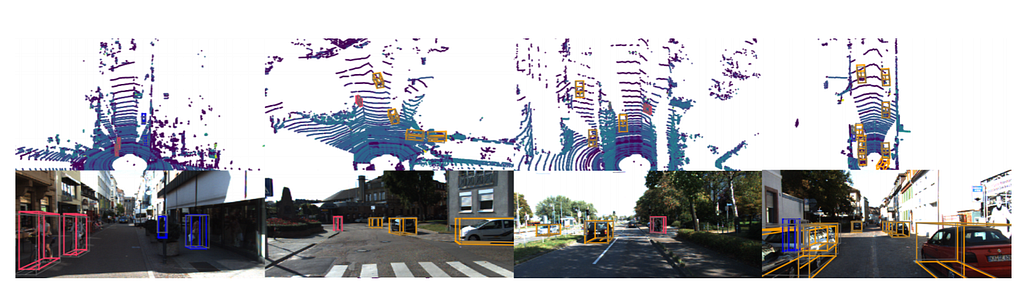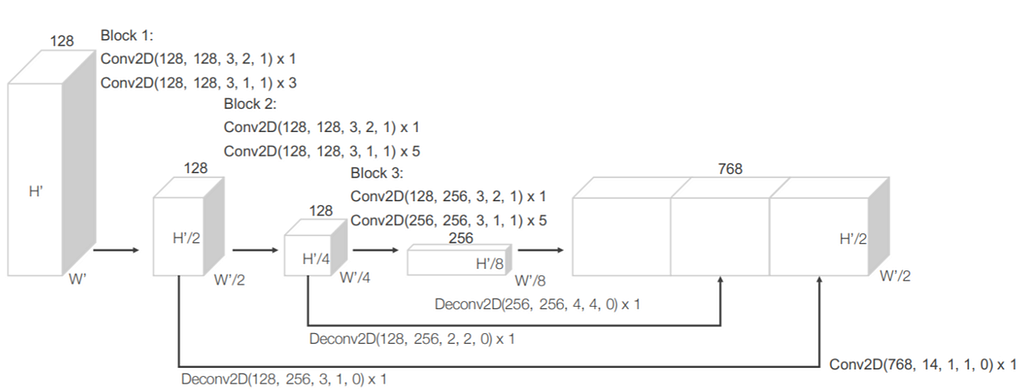# PointPillars — 3D point clouds bounding box detection and tracking (PointNet, PointNet++, LaserNet…

Original article was published by Anjul Tyagi on Becoming Human: Artificial Intelligence Magazine### PointPillars — 3D point clouds bounding box detection and tracking

Welcome to this multiple part series where we discuss five pioneering research papers for you to get started with 3D object detection. In this article, we will discuss PointPillars by Gregory P. Meyer et. al. Compared to the other works we discuss in this area, PointPillars is one of the fastest inference models with great accuracy on the publicly available self-driving cars dataset. PointPillars run at 62 fps which is orders of magnitude faster than the previous works in this area.

Feature Encoder (Pillar feature net): Converts the point cloud into a sparse pseudo image. First, the point cloud is divided into grids in the x-y coordinates, creating a set of pillars. Each point in the cloud, which is a 4-dimensional vector (x,y,z, reflectance), is converted to a 9-dimensional vector containing the additional information explained as follows:

• Xc, Yc, Zc = Distance from the arithmetic mean of the pillar c the point belongs to in each dimension.
• Xp, Yp = Distance of the point from the center of the pillar in the x-y coordinate system.

Hence, a point now contains the information D = [x,y,z,r,Xc,Yc,Zc,Xp,Yp].

From Pillars to a dense tensor (Stacked Pillars)

The set of pillars will be mostly empty due to sparsity of the point cloud, and the non-empty pillars will in general have few points in them. This sparsity is exploited by imposing a limit both on the number of non-empty pillars per sample (P) and on the number of points per pillar (N) to create a dense tensor of size (D, P, N). If a sample or pillar holds too much data to fit in this tensor the data is randomly sampled. Conversely, if a sample or pillar has too little data to populate the tensor, zero padding is applied. Note that D = [x,y,z,r,Xc,Yc,Zc,Xp,Yp] as explained in the previous section.

From Stacked Pillars to Learned Features

If you have been following up on this series, it is pretty clear that whenever we have to extract features from a point cloud type of data, we use PointNet.

PointNet basically applies to each point, a linear layer followed by BatchNorm and ReLU to generate high-level features, which in this case is of dimension (C,P,N). This is followed by a max pool operation which converts this (C,P,N) dimensional tensor to a (C,P) dimensional tensor.

### Trending AI Articles:

1. Microsoft Azure Machine Learning x Udacity — Lesson 4 Notes

2. Fundamentals of AI, ML and Deep Learning for Product Managers

4. Work on Artificial Intelligence Projects

Generating the Pseudo Image from Learned features

This is pretty straight-forward, the generated (C, P) tensor is transformed back to its original pillar using the Pillar index for each point. So originally, where the point was converted to a D dimensional vector, now it contains a C dimensional vector, which are the features obtained from a PointNet.

### BackboneAn example of a backbone (RPN) Region Proposal Network used in Point Pillars. The image is taken from the VoxelNet paper which originally proposed this network.

The backbone constitutes of sequential 3D convolutional layers to learn features from the transformed input at different scales. The input to the RPN is the feature map provided by the Feature Net. The architecture of this network is illustrated in the figure above. The network has three blocks of fully convolutional layers. The first layer of each block downsamples the feature map by half via convolution with a stride size of 2, followed by a sequence of convolutions of stride 1 (×q means q applications of the filter). After each convolution layer, BN and ReLU operations are applied. We then upsample the output of every block to a fixed size and concatenated to construct the high-resolution feature map.

ConvMD(cin, cout, k, s, p) to represent an M-dimensional convolution operator where cin and cout are the number of input and output channels, k, s, and p are the M-dimensional vectors corresponding to kernel size, stride size, and padding size respectively. When the size across the M-dimensions is the same, we use a scalar to represent the size e.g. k for k = (k, k, k).

This component is whole separate research that has been beautifully covered in this post. But the objective of the SSD network is to generate 2D bounding boxes on the features generated from the backbone layer of the Point Pillars network. Several important reasons for choosing SSD as a one-shot bounding box detection algorithm are:

• Fast inference.
• Uses features from well-studied networks like VGG.
• Great Accuracy.

They modify the original VGG network, which is simply the scaled-down part of the image above to concatenate features from different scales. SSD uses priors for regressing the bounding box locations and then Non-Maximum suppression to filter out noisy predictions. If this stuff appears to be fuzzy, I highly recommend peeking at this post and it won’t take you much time when you’ll understand all of it. Also, since SSD was originally developed for images, to modify the predictions for 3D bounding boxes, the height and elevation were made additional regression targets in the network.

### Results

In case you’re still in doubt about how good Point Pillars is, check out the results below. This thing is fast and very accurate, and the best part, it is built using existing networks and is an end to end trainable network.